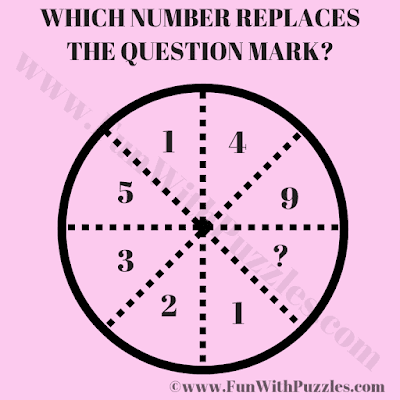This is the Logic Puzzle in Maths which will challenge your brain. In the given puzzle picture, you are shown some numbers inside the Circle. These numbers relate to each other Mathematically or/and logically. Your challenge is to deduce the logical reasoning hidden among the Circle numbers and then find the value of the missing number which will replace the question mark.Can you find the value of the missing number?
The answer to this "Logic Puzzle in Maths", can be viewed by clicking on the answer button.